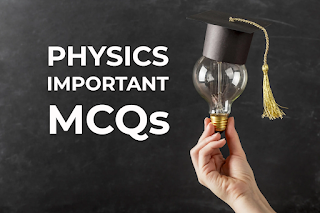# MDCAT Entry Test Preparation 2022 - MDCAT Physics Important MCQs 2022MDCAT Entry Test Preparation 2022 - MDCAT Physics Important MCQs 2022

1- A thermistor with a negative temperature coefficient is placed in a furnace. When the temperature of the furnace increases the resistance?    (UET 2015)
a) Decreases
b) Increases
c) Remains the same
d) No effect

2- A constant current of 1 ampere flows in an electrical component over a period of 5 seconds. The total charge flowing through the component over this duration is:   (UET 2016)
a) 5A
b) 10A
c) 15A
d) 20A

3- 2x10^6 electrons pass through a conductor in 1ms. Find the electric current flowing through the conductor:    (UHS 2017)
a) 32 x 10^-9 A
b) 32 x 10^-10 A
c) 3.2 x 10^-10 A
d) 3.2 x 10^-12 A

4- If e resistor having a resistance "R" is cut into three equal parts, then the equivalent of parallel combination is:     (UHS 2015)
a) 9/R
b) 3/R
c) R/9
d) R/3

5- Which combination of seven identical resistors each of 2 ohms gives rise to a resultant of 10/11 ohm?      (UHS 2015)
a) 5 parallel, 2 series
b) 4 parallel, 3 series
c) 2 parallel, 5 series
d) 3 parallel, 4 series

6- Energy consumed by a 60-watt bulb in 2 minutes is equal to:
a) 7.2 kilo-joules
b) 720 joules
c) 120 joules
d) 72000 joules

7- The magnetic flux linked with a solenoid of area 'A', having 'N' turns and a right angle to field 'B' is:
a) BA
b) 1/2 (NBA)
c) NBA
d) None is true

8- Magnetic field strength is measured in:
a) Wb/m
b) Wb/m^2
c) Wbm^2
d) Wb

9- Shunted galvanometer is called:
a) Ammeter
b) Voltmeter
c) Ohm meter
d) Potentiometer

10- An ideal voltmeter has:
a) zero ohm resistance
b) 100 ohms resistance
c) 1000 ohms resistance
d) Infinite resistance

11- Which one of the following is not an accurate measuring device?
a) Digital multimeter
b) CRO
c) Voltmeter
d) Potentiometer

12- Which one of the following has the least resistance?
a) Galvanometer
b) Ammeter
c) Voltmeter
d) Ohm meter

13- A transformer has 100 turns on the input side and 500 turns on the output side. If the RMS value of input voltage and current is 220V and 5A respectively. The output power is:   (UET 2015)
a) 500 watt
b) 1100 watt
c) 1440 watt
d) 50 watt

14- Lenz's law in electromagnetic induction is the direct consequence of the principle of conversation of: (UHS 2018)
a) Energy
b) Charge
c) Momentum
d) Mass

15- The cause of induced emf is:     (UHS 2017)
a) Rate of change of magnetic flux
b) Change in magnetic flux
c) Increase in magnetic flux
d) Decrease in magnetic flux

16- The range of visible wavelength spectrum is:      (UET 2010)
a) 400-750
b) 100-400
c) 400-1000
d) 300-600

17- When the effective value of current is 10. What is its peak value?      (UET 2010)
a) 12
b) 14.2
c) 10.5
d) 13

18- The peak value of an alternating 60 Hz power supply is 140V. An A.C. voltmeter connected to the power supply will give a reading of approximately:          (ECAT 2019)
a) 100V
b) 198V
c) 140V
d) 70V

19- The modulus of elasticity depends upon (UHS 2017)
a) Nature of the material
b) Temperature
c) Both a and b
d) Area of cross-section

20- A 1.25 cm diameter cylinder is subjected to a load of 2500 kg. Calculate the stress on the bar:      (UHS 2013)
a) 2 x 10^2 Pa
b) 200 Pa
c) 200 x 10^6 Pa
d) 2 x 10^6 Pa